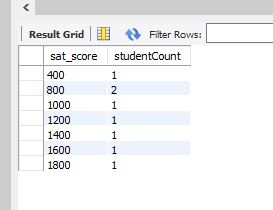# SQL COUNT Aggregate Function

SQL COUNT Aggregate Function
0

The COUNT operator is usually used in combination with a GROUP BY clause. It is one of the SQL “aggregate” functions, which include AVG (average) and SUM.

This function will count the number of rows and return that count as a column in the result set.

Here are examples of what you would use COUNT for:

• Counting all rows in a table (no group by required)
• Counting the totals of subsets of data (requires a Group By section of the statement)

For reference, here is the current data for all the rows in our example student database.

``````select studentID, FullName, programOfStudy, sat_score from student; -- all records with fields of interest
``````This SQL statement provides a count of all rows. Note that you can give the resulting COUNT column a name using “AS”.

``````select count(*) AS studentCount from student; -- count of all records
``````Here we get a count of students in each field of study.

`````` select studentID, FullName, count(*) AS studentCount from the student table with a group by programOfStudy;
``````Here we get a count of students with the same SAT scores.

`````` select studentID, FullName, count(*) AS studentCount from the student table with a group by sat_score;
``````Here is an example using the campaign funds table. This is a sum total of the dollars in each transaction and the number of contributions for each political party during the 2016 US Presidential Campaign.

``````select Specific_Party, Election_Year, format(sum(Total_\$),2) AS contribution\$Total, count(*) AS numberOfContributions
from combined_party_data
group by Specific_Party,Election_Year
having Election_Year = 2016;
``````As with all of these things there is much more to it, so please see the manual for your database manager and have fun trying different tests yourself.